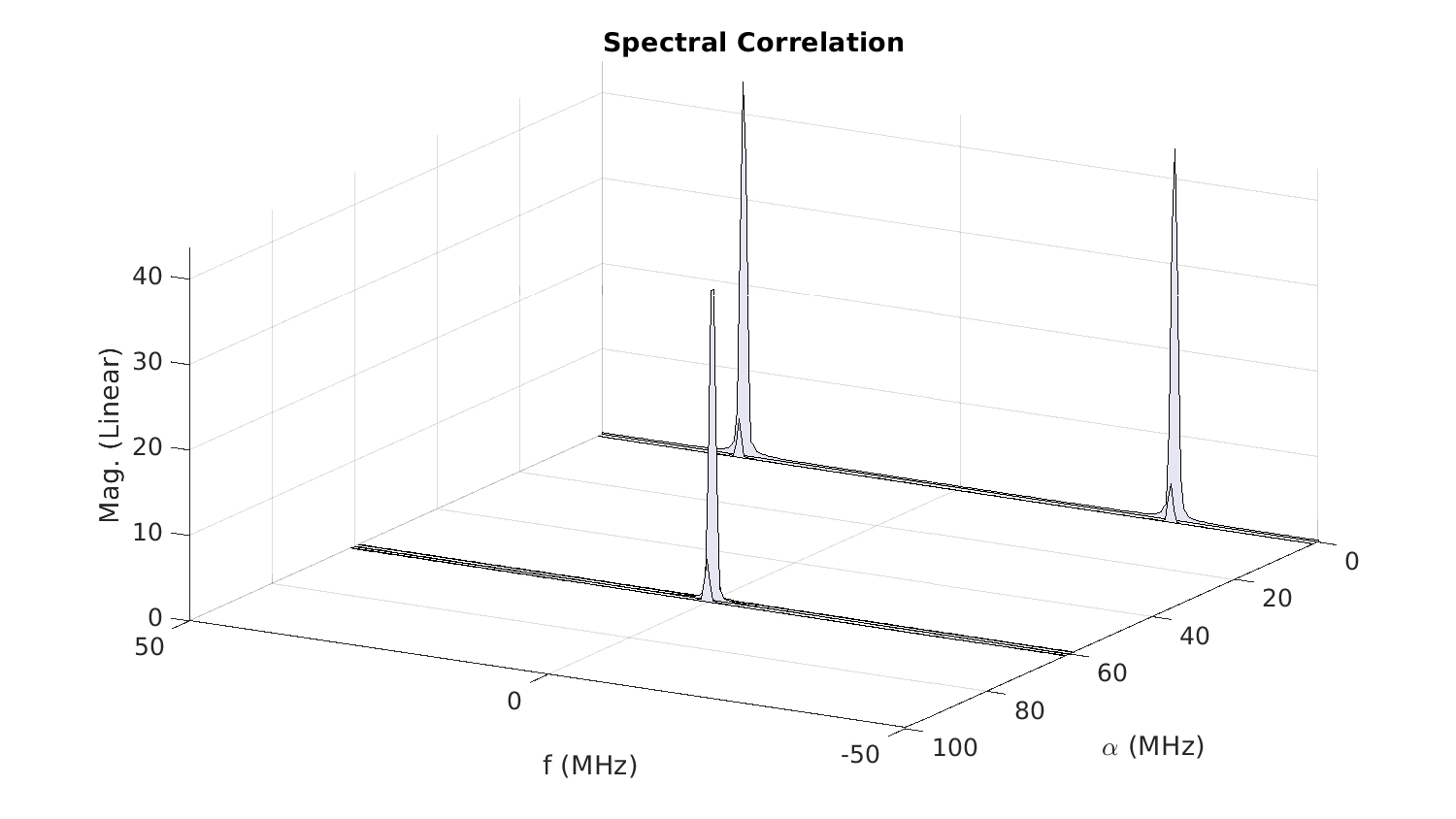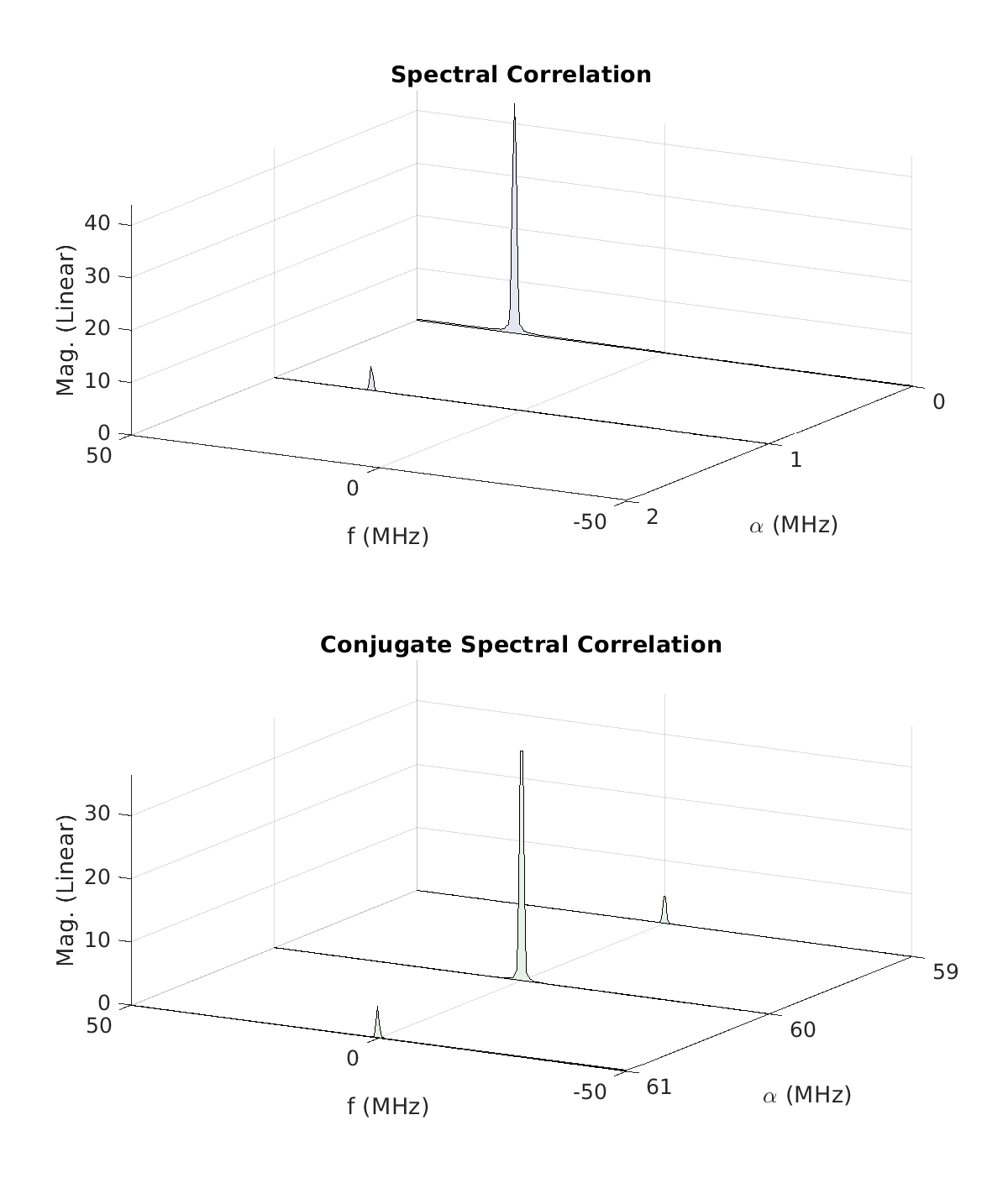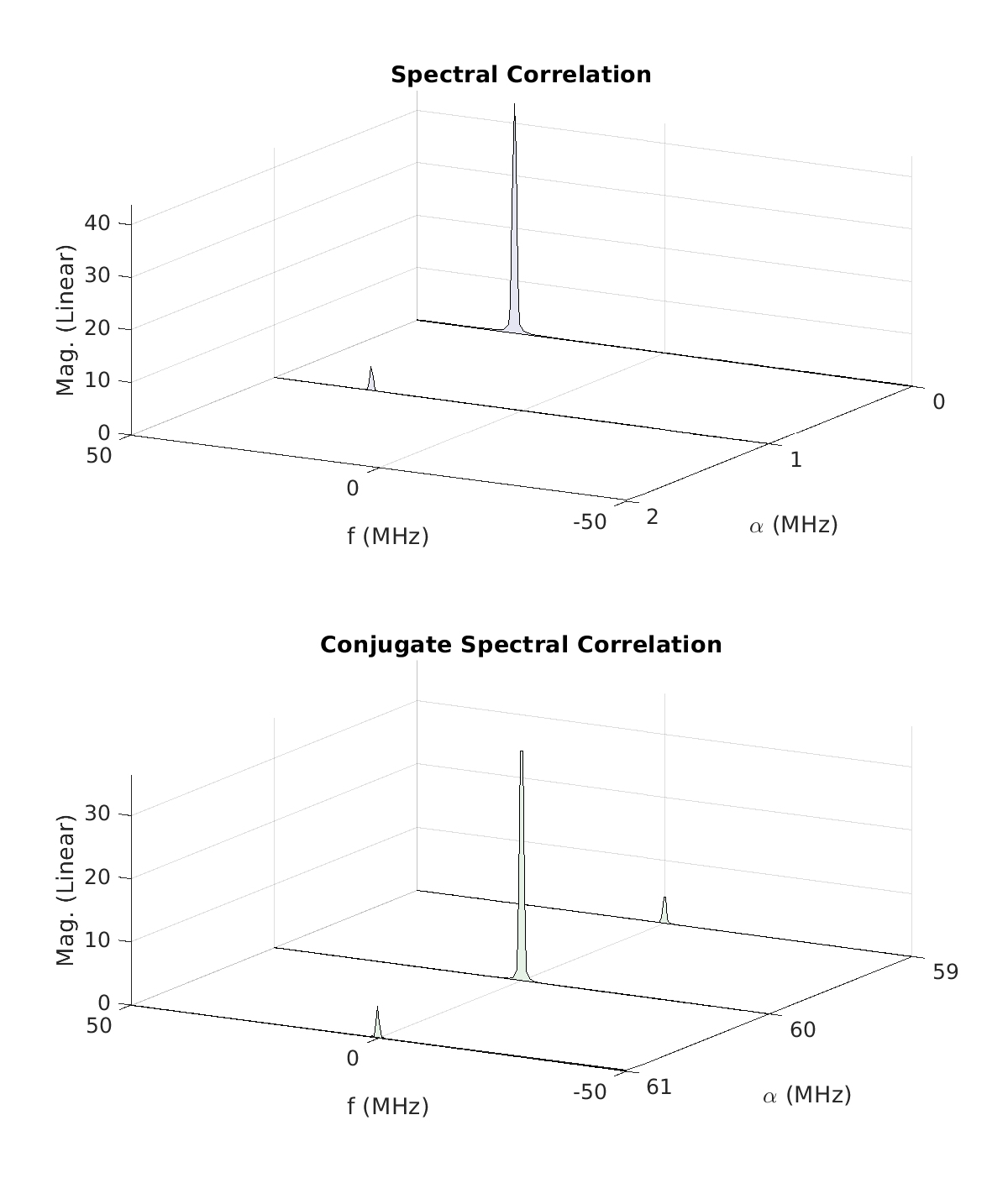# SPTK: The Analytic Signal and Complex Envelope

In signal processing, and in CSP, we often have to convert real-valued data into complex-valued data and vice versa. Real-valued data is in the real world, but complex-valued data is easier to process due to the use of a substantially lower sampling rate.

Previous SPTK Post: The Moving-Average Filter    Next SPTK Post: Random Variables

In this Signal-Processing Toolkit post, we review the signal-processing steps needed to convert a real-valued sampled-data bandpass signal to a complex-valued sampled-data lowpass signal. The former can arise from sampling a signal that has been downconverted from its radio-frequency spectral band to a much lower intermediate-frequency spectral band. So we want to convert such data to complex samples at zero frequency (‘complex baseband’) so we can decimate them and thereby match the sample rate to the signal’s baseband bandwidth. Subsequent signal-processing algorithms (including CSP of course) can then operate on the relatively low-rate complex-envelope data, which is beneficial because the same number of seconds of data can be processed using fewer samples, and computational cost is determined by the number of samples, not the number of seconds.

[Jump straight to ‘Significance of the Complex Envelope in CSP’ below.]

Let’s start at the beginning, and when we come to the end, stop. Any radio-frequency signal can be represented by the following formula$\displaystyle x_{RF}(t) = \Re \left[ x_{BB}(t) e^{i 2 \pi f_c t} \right], \hfill(1)$

where$x_{BB}(t)$ is a complex-valued signal, bearing the message to be transmitted in the case of communications, or bearing a sensing waveform, in the case of radar and other sensing signals. The frequency$f_c$ is the carrier frequency (or center frequency) and it is chosen so that the overall signal$x_{RF}(t)$ has its energy concentrated in frequency near$f_c$, which is a band of frequencies for which it is known that the targeted physical signal-propagation medium has relatively low attenuation. That is, the physical medium, viewed as a linear time-invariant system, has a passband that includes$f_c$ and nearby frequencies.

The signal$x_{BB}(t)$ is a lowpass signal that is referred to as the complex baseband signal, or as a complex envelope. It is a fictitious signal, being complex-valued, but the real and imaginary parts of the signal are themselves actual signals and can be constructed in physical circuits starting with the message bits (digital message) or message voltage (analog message) to be conveyed across the channel to a receiver (communications) or target (radar).

RF receiving equipment can frequency-translate real-world signals like$x_{RF}(t)$, resulting in other real-valued real-world signals but with significantly reduced carrier frequency. When this reduced carrier frequency is close enough to zero, so that the signal can be adequately sampled with physical real-world analog-to-digital (ADC) converters, the carrier frequency is referred to as an intermediate frequency (IF),$\displaystyle x_{IF}(t) = \Re \left[ x_{BB}(t) e^{i 2 \pi f_{IF} t + i\theta_0} \right]. \hfill (2)$

Here$f_{IF} \ll f_c$ is the intermediate frequency (intermediate between a high carrier frequency, which is the RF signal’s center frequency, and zero, which is the baseband signal’s center frequency).

So it is often the case that one is provided a sampled version of (2), or one has access to equipment that provides (2) itself in continuous time, and has control over a suitable sampling device that follows.

The question is how to convert the sampled real-valued intermediate-frequency signal$x_{IF}(t)$ to a sampled complex-valued baseband-frequency signal. This is an important question because we want to work with the random signal represented by$x_{BB}(t)$ for a variety of reasons (detection, parameter estimation, direction finding, demodulation, signal separation, synchronization, channel estimation, modulation recognition). And for any signal-processing algorithm that requires$T$ seconds of data for adequate performance, we want to work with the minimum number of samples of the signal to minimize computational costs (minimize multiplications and additions in a digital computer). So we want to work with all the information in$x_{BB}(t)$ but at the lowest sampling rate possible. That means we need a lowpass signal according to the sampling theorem. (I know this is an oversimplified view of the situation, since one can play all sorts of tricks with low-rate sampling of high-frequency signals, cleverly placing one of the aliases created by the sampling into a frequency band that can be easily accessed.)

### Step-by-Step Example

Let’s consider a real-valued sampled-data IF signal that is a BPSK signal (of course!) in noise. The sampling rate is$100$ MHz,$f_{IF}$ is$30$ MHz, and the symbol rate of the BPSK signal is$1$ MHz. So this is just a straightforward real-world sampling of a real-world electromagnetic wave resulting in some giant vector of floating-point numbers–no complex-number funny business (yet). A power spectrum estimate for the real-valued IF data is shown in the top plot of Figure 1. Because the data is real-valued, we see the BPSK PSD peak at both$+30$ MHz and$-30$ MHz.Figure 1. Three related power spectral densities. Upper: a real-valued sample-data BPSK signal in noise. Middle: The analytic signal for the data in the upper plot. Lower: the complex envelope (baseband signal) for the signal in the upper plot using an assumed center frequency of 25 MHz.

The analytic signal part of the RF signal is the part of the signal that corresponds to positive frequencies. If the bandwidth of the signal$x_{BB}(t)$ is much smaller than the intermediate frequency$f_{IF}$, then we can identify the analytic signal as$\displaystyle x_{AS}(t) = \frac{1}{2} x_{BB}(t) e^{i2\pi f_{IF} t + i \theta_0} \hfill (3)$

because$\displaystyle \Re [z] = \frac{1}{2} (z + z^*). \hfill (4)$

The analytic signal for our BPSK example is shown in the middle plot of Figure 1. To obtain the analytic signal from provided real-valued data, we can Fourier transform the data and set all Fourier-transform values for negative frequencies to zero, then inverse transform. Because we have broken the symmetry in the transform that exists for real-valued time-domain signals, that last inverse Fourier transform will result in complex-valued data, so now we have a complex-valued representation of the real-valued intermediate-frequency data.

But the analytic signal, as defined here, is still sampled at the same rate as is the intermediate-frequency signal, which is generally (but not always) much greater than the sampling rate that is implied by the bandwidth of the signal(s) within the positive-frequency portion of the IF-signal spectrum. So we’ve not gained anything yet. But we’ve not lost any information either, because we can reverse our steps and get back to the original real-valued signal.

The next step is to frequency translate the analytic signal to zero frequency–shift it to the left by$f_s/4$ Hz:$\displaystyle y(t) = x_{AS}(t) e^{i2\pi (-f_s/4) t}. \hfill (5)$

Then to compute the basic complex envelope signal, decimate this frequency-shifted signal by two. The result is shown in the lower plot of Figure 1. By keeping track of the signal-processing steps, I can compute the correct values of frequency with which to label the x-axis.

But let’s get to the connection to CSP by looking at the spectral correlation function for each of the signals in Figure 1 (the original real-valued IF signal, the analytic signal, and the complex-envelope signal). I’ve estimated the non-conjugate and conjugate spectral correlation functions for each of these signals and plotted them as surfaces in my usual style:Figure 2. The spectral correlation magnitude for the real-valued sampled-data intermediate-frequency signal with PSD shown in the top plot of Figure 1. Since this signal is real-valued, the non-conjugate and conjugate surfaces are identical, so we show only one. Moreover, the symmetries in cycle frequency for non-conjugate spectral correlation functions apply, so we’ve not plotted the surface for negative cycle frequencies.Figure 3. The spectral correlation surfaces for the analytic signal with PSD shown in the middle plot of Figure 1. Since this signal is complex-valued, we show both the non-conjugate and conjugate spectral correlation surfaces, which differ.Figure 4. The spectral correlation surfaces for the complex-envelope signal with PSD shown in the lower plot of Figure 1. This signal differs from the analytic signal in Figure 3 by a frequency shift and a decimation-by-two. So the BPSK signal’s PSD is near zero frequency and the sampling rate is half that of the analytic signal.

We don’t need to retain all the spectral components for positive frequencies when we create the analytic signal. We can focus on any subset, such as a much more narrow band of frequencies that contain the energy of a signal or signals of interest.

For example, we can retain$2.5$ MHz of bandwidth centered at$30.5$ MHz instead of$50$ MHz centered at$25$ MHz as in Figure 1. Notice that I’m choosing a center frequency that does not exactly coincide with the center frequency of the BPSK signal ($30$ MHz). This choice of a center frequency for analytic-signal creation, and therefore for the frequency shift in creating the complex-envelope signal from the analytic signal, will result in the BPSK signal appearing at a smallish frequency offset from zero in the final complex envelope. This is the origin of the carrier offset frequency we’ve discussed elsewhere. The resulting sequence of PSD estimates is shown in Figure 5.Figure 5. Power spectrum estimates for the original real-valued intermediate-frequency signal (upper), analytic signal with center frequency 30.5 MHz and bandwidth 2.5 MHz (middle), and the complex-envelope signal formed by frequency shifting the analytic signal by 30.5 MHz and subsampling by a factor of 100/2.5 = 40 (lower).

The spectral correlation function for the intermediate-frequency signal with PSD shown in the upper plot of Figure 5 is the same as that for Figure 1, so we show only the spectral correlation functions for the analytic signal and complex envelope next:Figure 6. Spectral correlation surfaces for the complex-valued analytic signal with PSD shown in the middle plot of Figure 5.Figure 7. The spectral correlation surfaces for the complex-envelope signal with PSD shown in the lower plot of Figure 5. Finally here we have arrived at a signal that has sampling rate reasonably close to the Nyquist sampling rate implied by the bandwidth of the BPSK signal.

I’ve plotted the spectral correlation surfaces for the two complex envelopes as if the center frequency for the data is zero, but scaled the frequency and cycle frequency axes using the actual sampling rate. With this style of plotting, the absolute IF frequency for the BPSK signal in the original IF data is lost. But if desired you can add it back into the appropriate axes for the plots if desired. For the non-conjugate surface in Figure 7, we know that$f=0$ corresponds to a physical frequency of$f = 30.5$ MHz, because that’s the center frequency we used to shift the analytic signal. So we would add$30.5$ MHz to the shown$f$-axis values, which would put the center frequency for the BPSK signal at$30.5 + (-0.5) = 30$ MHz, which we know is correct. Similarly, for the conjugate surface in Figure 7, we would add$2*(30.5) = 61$ MHz to the cycle-frequency axis, which would place the doubled-carrier feature at$61 + (-1) = 60$ MHz, which is also correct for our BPSK signal with carrier frequency of$30$ MHz.

### Significance of Complex Envelopes in CSP

Most signal processors (meaning people, not devices) use complex-valued data because it is a convenient way to represent an actual RF signal, which is real-valued, without having to use a high sample rate as would be dictated by the carrier frequency of the RF signal. The drawback in CSP is that we then need to consider two kinds of spectral correlation function to fully relate the cyclostationarity of the complex envelope to the cyclostationarity of the real RF signal. This is explained in some detail in the post on conjugation configurations.

Previous SPTK Post: The Moving-Average Filter Next SPTK Post: Random Variables